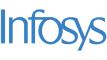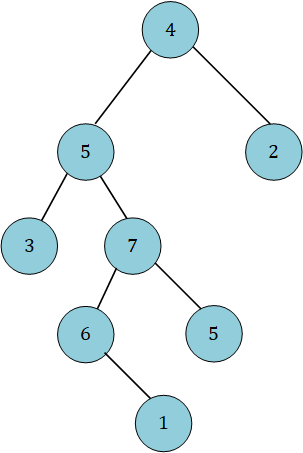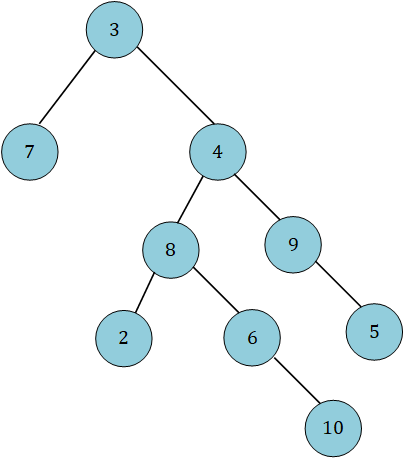New update is available. Click here to update.
Topics

# Lowest Common Ancestor of a Binary Tree III

Moderate0/80
Average time to solve is 25m+5 more companies

## Problem statement

The node structure of a binary tree is modified such that each node has the reference to its parent node.

You are given two nodes: ‘N1’ and ‘N2’, of the above binary tree. Your task is to return the lowest common ancestor (LCA) of the given nodes.

Note:

``````Let ‘TREE’ be a binary tree. The lowest common ancestor of two nodes, ‘N1’ and ‘N2’, is defined as the lowest node in ‘TREE’ with ‘N1’ and ‘N2’ as descendants (where we allow a node to be a descendant of itself).
``````
Detailed explanation ( Input/output format, Notes, Images )
Constraints :
``````1 <= T <= 100
1 <= N <= 10 ^ 4
1 <= DATA <= 10 ^ 4
N1 != N2
N1 and N2 exist in the ‘TREE’.
The ‘TREE’ contains unique nodes.

Where ‘T’ is the number of test cases, ‘N’ is the number of nodes in the ‘TREE’, ‘DATA’ represents the value of the node, ‘N1’ and ‘N2’ represent the nodes of which LCA has to be found.

Time limit: 1 sec.
``````

#### Sample Input 1:

``````2
3 1
4 5 2 3 7 -1 -1 -1 -1 6 5 -1 1 -1 -1 -1 -1
2 10
3 7 4 -1 -1 8 9 2 6 -1 5 -1 -1 -1 10 -1 -1 -1 -1
``````

#### Sample Output 1:

``````5
8
``````

#### Explanation of Sample Output 1:

``````Test Case 1 :
````````````For nodes 3 and 1, common ancestors are 5 and 4. Out of which, 5 is the nearest ancestor to both nodes. So, LCA(3, 1) = 5.

Test Case 2 :
````````````For nodes 2 and 10, common ancestors are 8, 4 and 3. Out of which, 8 is the nearest ancestor to both nodes. So, LCA(2, 10) = 8.
``````

#### Sample Input 2:

``````2
6 4
2 1 6 -1 -1 4 -1 -1 -1
3 5
3 -1 4 -1 5 -1 -1
``````

#### Sample Output 2:

``````6
3
``````Console